# Live oaks introgression manuscript¶

## Notebook 4: Population analyses¶

#### D. Eaton, A. Hipp, A. Gonzalez-Rodriguez & J. Cavender-Bares¶

##### contact: [email protected]¶

In :
%%bash
mkdir -p analysis_treemix/
mkdir -p analysis_structure/

In :
import numpy as np
import gzip
from collections import OrderedDict, Counter

import matplotlib.pyplot as plt
import seaborn as sns
%matplotlib inline


## Treemix analysis¶

I run a custom python code below to convert the unlinked snps file from the pyrad output into a Treemix input file. This requires grouping sampled individuals into populations, and calling biallelic SNP frequencies for them. I grouped the four non-Virentes white oaks into the outgroup sample. Each ingroup taxon is its own population, except for Q. fusiformis for which I split up the Texas and Mexico populations, for a total of 9 populations.

In :
## group sample names into taxon names
## again I exclude the South Carolina sample of virginiana, which has less data.

taxa = {
'virginiana' : ["LALC2","FLSF33","FLBA140"],
'minima'     : ["FLCK216","FLSA185","FLMO62","FLSF47"],
'geminata'   : ["FLCK18","FLWO6","FLSF54","FLAB109"],
'sagraeana'  : ["CUSV6","CUVN10","CUCA4"],
'oleoides'   : ["MXSA3017","BZBB1","HNDA09","CRL0001","CRL0030"],
'brandegeei' : ["BJSB3","BJSL25","BJVL19"],
'fusiformis.m' : ["MXED8","MXGT4"],
'fusiformis.t' : ["TXMD3","TXGR3"],
'outgroup'   : ["EN","AR","DO","DU"],
}

## used to require that all populations have data for at least
## one individual in each locus that we use
minhits = *9

In :
## resolve ambiguity characters
def resolve(base):
D = {'R':['G','A'],
'K':['G','T'],
'S':['G','C'],
'Y':['T','C'],
'W':['T','A'],
'M':['C','A'],}
if base in D:
return D[base]
else:
return [base,base]

In :
## print groups and minhits
for i,j in zip(taxa,minhits):
print "\t   ",i, taxa[i], 'minimum=',j

## read in data to sample names
nsamp,nsnps = dat.strip().split(" ")
nsamp = int(nsamp)
nsnps = int(nsnps)
NDATA = np.empty([int(nsamp),int(nsnps)],dtype='object')

## read SNP matrix into a numpy.array
for line in range(len(dat[1:])):
a,b = dat[1:][line].split()
NDATA[line] = list(b)
sites = np.transpose(NDATA)

## unpack ambiguity bases and find two most common alleles
## at every SNP site, save to a list
alleles = []
for site in sites:
ds = []
for s in site:
if s in list("RKSYWM"):
ds.append(resolve(s))
ds.append(resolve(s))
else:
ds.append(s)
ds.append(s)
snp = [s for s in ds if s not in ["N",'-']]
a = Counter(snp).most_common(3)
alleles.append([a,a])

## create a dictionary mapping sample names to SNPs
SNPS = OrderedDict()
for line in dat[1:]:
a,b = line.split()
SNPS[a] = b

## create a dictionary with empty lists for each taxon
FREQ = OrderedDict()
for tax in taxa:
FREQ[tax] = []

## fill the FREQ dictionary with SNPs for all
## samples in that taxon
keeps = []
for snp in range(int(nsnps)):
GG = []
for tax,mins in zip(taxa,minhits):
GG.append( sum([SNPS[i][snp] not in ["N","-"] for i in taxa[tax]]) >= int(mins))
if all(GG):
keeps.append(snp)

for keep in keeps:
for tax in FREQ:
bunch = []
for i in taxa[tax]:
#print tax, i, SNPS[i][keep]
bunch.append(resolve(SNPS[i][keep]))
bunch.append(resolve(SNPS[i][keep]))
FREQ[tax].append("".join(bunch))

## output files
outfile = gzip.open("analysis_treemix/treemix_SNPs.gz",'w')

print >>outfile, " ".join(FREQ.keys())

## print data
for i,j in enumerate(keeps):
a1 = alleles[j]
a2 = alleles[j]
H = [str(FREQ[tax][i].count(a1))+","+str(FREQ[tax][i].count(a2)) for tax in FREQ]

## exclude non-biallelic SNPs
if "0,0" not in " ".join(H):
## exclude if not variable in subsampling
if not all([i.split(",") == '0' for i in H]):
print >>outfile, " ".join(H)
outfile.close()

	    geminata ['FLCK18', 'FLWO6', 'FLSF54', 'FLAB109'] minimum= 1
minima ['FLCK216', 'FLSA185', 'FLMO62', 'FLSF47'] minimum= 1
fusiformis.m ['MXED8', 'MXGT4'] minimum= 1
brandegeei ['BJSB3', 'BJSL25', 'BJVL19'] minimum= 1
outgroup ['EN', 'AR', 'DO', 'DU'] minimum= 1
oleoides ['MXSA3017', 'BZBB1', 'HNDA09', 'CRL0001', 'CRL0030'] minimum= 1
virginiana ['LALC2', 'FLSF33', 'FLBA140'] minimum= 1
fusiformis.t ['TXMD3', 'TXGR3'] minimum= 1
sagraeana ['CUSV6', 'CUVN10', 'CUCA4'] minimum= 1

In :
! zcat analysis_treemix/treemix_SNPs.gz | wc

  12061  108549  434320

In :
%%bash
treemix -i analysis_treemix/treemix_SNPs.gz -root outgroup -k 1      -o analysis_treemix/SNPs_m0 > analysis_treemix/logSm0
treemix -i analysis_treemix/treemix_SNPs.gz -root outgroup -k 1 -m 1 -o analysis_treemix/SNPs_m1 > analysis_treemix/logSm1
treemix -i analysis_treemix/treemix_SNPs.gz -root outgroup -k 1 -m 2 -o analysis_treemix/SNPs_m2 > analysis_treemix/logSm2
treemix -i analysis_treemix/treemix_SNPs.gz -root outgroup -k 1 -m 3 -o analysis_treemix/SNPs_m3 > analysis_treemix/logSm3
treemix -i analysis_treemix/treemix_SNPs.gz -root outgroup -k 1 -m 4 -o analysis_treemix/SNPs_m4 > analysis_treemix/logSm4
treemix -i analysis_treemix/treemix_SNPs.gz -root outgroup -k 1 -m 5 -o analysis_treemix/SNPs_m5 > analysis_treemix/logSm5
treemix -i analysis_treemix/treemix_SNPs.gz -root outgroup -k 1 -m 6 -o analysis_treemix/SNPs_m6 > analysis_treemix/logSm6
treemix -i analysis_treemix/treemix_SNPs.gz -root outgroup -k 1 -m 7 -o analysis_treemix/SNPs_m7 > analysis_treemix/logSm7


## Plot the treemix results¶

In :
%load_ext rmagic
## this requires that you have the python module rpy2 installed

In :
%%R
source("~/local/src/treemix/src/plotting_funcs.R")

In :
%%bash
echo -e "virginiana\nminima\ngeminata\nsagraeana\noleoides\nfusiformis.m\nfusiformis.t\nbrandegeei\noutgroup" >analysis_treemix/poporder

In :
%%R -h 400 -w 800
## save figure
#svg("figures/treemix_m0.svg", width=8, height=4)
par(mfrow=c(1,2));
plot_tree("analysis_treemix/SNPs_m0", lwd=3)
plot_resid("analysis_treemix/SNPs_m0","analysis_treemix/poporder")
#dev.off()

 "mse 0.000551605086419753"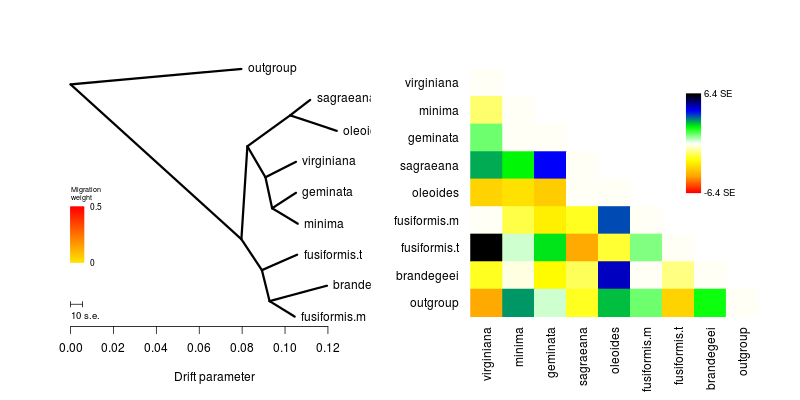In :
%%R -h 400 -w 800
## save figure
#svg("figures/treemix_m1.svg", width=8, height=4)
par(mfrow=c(1,2));
plot_tree("analysis_treemix/SNPs_m1", lwd=3)
plot_resid("analysis_treemix/SNPs_m1","analysis_treemix/poporder")
#dev.off()

 "mse 0.000551605086419753"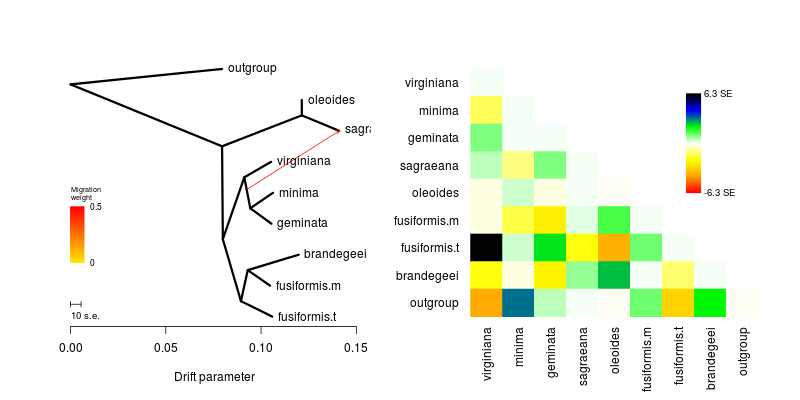In :
%%R -h 400 -w 800
## save figure
#svg("figures/treemix_m2.svg", width=8, height=4)
par(mfrow=c(1,2));
plot_tree("analysis_treemix/SNPs_m2", lwd=3)
plot_resid("analysis_treemix/SNPs_m2","analysis_treemix/poporder")
#dev.off()

 "mse 0.000551605086419753"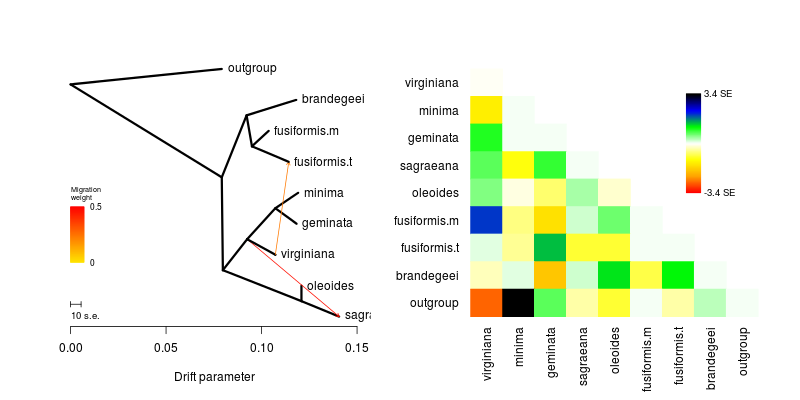In :
%%R -h 400 -w 800
## save figure
par(mfrow=c(1,2));
plot_tree("analysis_treemix/SNPs_m3", lwd=2)
plot_resid("analysis_treemix/SNPs_m3","analysis_treemix/poporder")

 "mse 0.000551605086419753"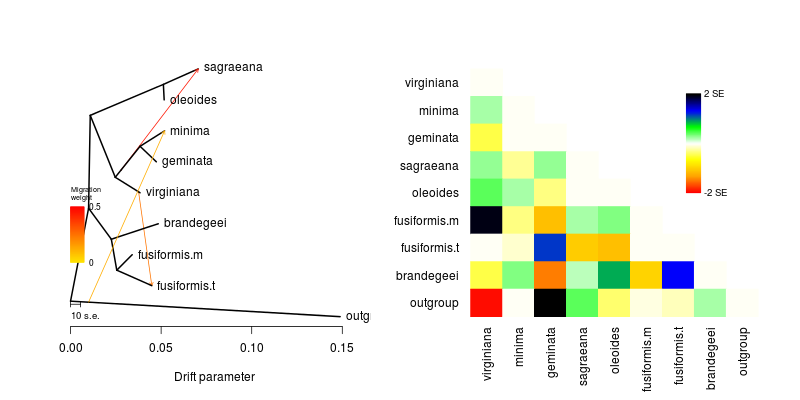In :
%%R -h 400 -w 800
## save figure
par(mfrow=c(1,2));
plot_tree("analysis_treemix/SNPs_m4", lwd=2)
plot_resid("analysis_treemix/SNPs_m4","analysis_treemix/poporder")

 "mse 0.000551605086419753"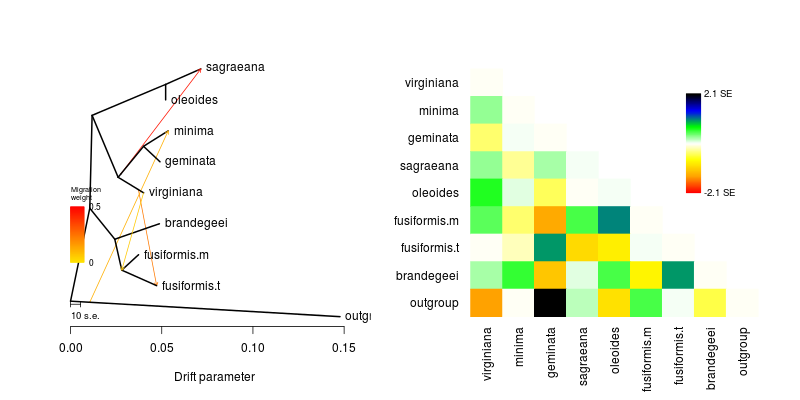In :
%%R -h 400 -w 800
## save figure
par(mfrow=c(1,2));
plot_tree("analysis_treemix/SNPs_m5", lwd=2)
plot_resid("analysis_treemix/SNPs_m5","analysis_treemix/poporder")

 "mse 0.000551605086419753"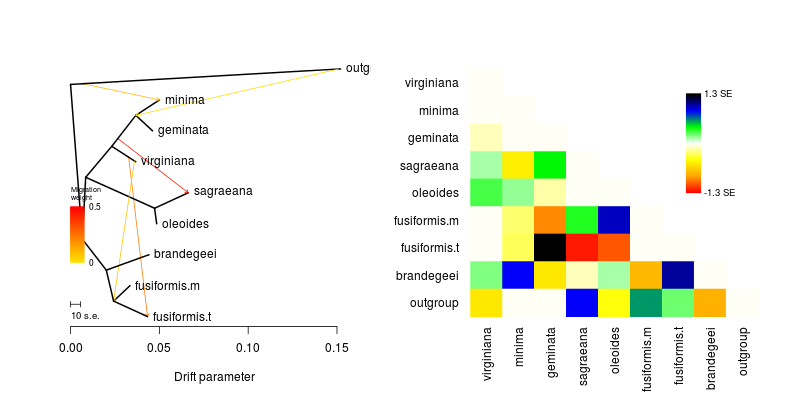## Structure analysis of RADseq SNPs¶

Structure was run on the structure formatted ('k' option) SNP output from pyRAD in the file "virentes_c85d6m20p5noutg.str". Clustering assignment was measured for K values between 2-8, and the optimal K value was inferred by Evanno's K, as described in the text. Tthe optimal K value is 7. Here we run 10 replicate analyses at each value of K, and estimate average cluster assignment values by permuting across replicates using CLUMPP, and finally plot the results as a stacked barplot.

In [ ]:
## download fresh (default) params files (or you could get them elsewhere)

In [ ]:
## set the file format to match pyRAD structure format (6 blank columns)
## meaning no populations, locations, names, or phenotypes are indicated.
stderr = ! sed -i '/LABEL /c\#define LABEL 1 //'         analysis_structure/struct.main
stderr = ! sed -i '/POPDATA /c\#define POPDATA 0 //'     analysis_structure/struct.main
stderr = ! sed -i '/POPFLAG /c\#define POPFLAG 0 //'     analysis_structure/struct.main
stderr = ! sed -i '/LOCDATA /c\#define LOCDATA 0 //'     analysis_structure/struct.main
stderr = ! sed -i '/PHENOTYPE /c\#define PHENOTYPE 0 //' analysis_structure/struct.main
stderr = ! sed -i '/EXTRACOLS /c\#define EXTRACOLS 0 //' analysis_structure/struct.main
stderr = ! sed -i '/BURNIN /c\#define BURNIN 50000 //'     analysis_structure/struct.main
stderr = ! sed -i '/NUMREPS /c\#define NUMREPS 500000 //'   analysis_structure/struct.main

stderr = ! sed -i '/RANDOMIZE /c\#define RANDOMIZE 0 //'   analysis_structure/struct.extras
stderr = ! sed -i '/LOCISPOP /c\#define LOCISPOP 0 //'     analysis_structure/struct.extras
stderr = ! sed -i '/USEPOPINFO /c\#define USEPOPINFO 0 //' analysis_structure/struct.extras

In [ ]:
## create a Parallel computing client
## can map a function across how ever many
## parallel engines are turned on in the notebook
## under the Clusters tab
from IPython.parallel import Client
PAR = Client()[:]

In [ ]:
## decorator adds parallel capability if run in IPython notebook
def structure(arg):
ff = arg
NUMINDS, NUMLOCI = (27,14011)
cmd = """ -m analysis_structure/struct.main \
-e analysis_structure/struct.extras \
-K %d \
-N %s \
-L %s \
-i %s.str \
-D %s \
-o %s """ % (arg, NUMINDS, NUMLOCI, ff, str(arg), arg+".K"+str(arg))
stderr = ! structure $cmd  In [ ]: args = [[K, "analysis_pyrad/outfiles/virentes_c85d6m20p5noutg", "analysis_structure/RADrep"+str(reps), np.random.randint(0,1e9,1)] for K in range(2,9) \ for reps in range(1,11)] for i in args[:]: print i  In [ ]: with open("analysis_pyrad/outfiles/virentes_c85d6m20p5noutg.str",'r') as ifile: print len(ifile.readline().strip().split()), 'SNPs in structure data set'  In [ ]: ## run these jobs in parallel quiet = structure.map(args)  ### Use CLUMPP to get means across permuted replicates¶ First I create concatenated indfiles by cutting out the relevant lines of the STRUCTURE output and appending to a single file for all replicates with the same value of$K$. Then I create and edit a CLUMPP params file, entering settings to perform permutations of all iterations using the greedy algorithm. In [ ]: def run_CLUMPP(K): repfiles = glob.glob("analysis_structure/RADrep*.K"+str(K)+"_f") outfile = open("analysis_structure/RADr"+str(K)+".indfile",'w') for ff in repfiles: i = 1 for line in open(ff).readlines(): if ") : " in line: ll = line.strip().split() print >>outfile, " ".join([ll,ll,ll,ll.split('.')])+" : "+" ".join(ll[4:]) i += 1 print >>outfile, "" outfile.close() ## get template CLUMPP params file, I host a template file at this download ! wget -q www.dereneaton.com/downloads/clumpp.paramfile -O analysis_structure/clumpp.paramsfile ## fill in the template: tell it the format to use individuals stderr = ! sed -i '/type of data /c\DATATYPE 0 # individuals //' analysis_structure/clumpp.paramsfile stderr = ! sed -i '/individuals or populations /c\C 27 # N individuals //' analysis_structure/clumpp.paramsfile stderr = ! sed -i '/Print the permuted /c\PRINT_PERMUTED_DATA 0 # no print //' analysis_structure/clumpp.paramsfile ## call CLUMPP NREPS = 10 NINDS = 27 args = "-i analysis_structure/RADr%s.indfile \ -o analysis_structure/RADr%s.outfile \ -j analysis_structure/RADr%s.miscfile \ -k %s -r %s -c %s " % (K,K,K,K,NREPS,NINDS) stderr = ! ~/local/src/CLUMPP_Linux64.1.1.2/CLUMPP analysis_structure/clumpp.paramsfile$args

In [ ]:
## run CLUMPP over 10 iterations at each level of K
for i in range(2,9):
run_CLUMPP(i)


## Make a stacked barplot¶

In :
def structplot(infile, K, colorder=[], barorder=[]):
D = OrderedDict()
for line in dat:
ll = line.strip().split()
vals = [float(i)*100 for i in ll[5:]]

## order of the bars
if not barorder:
D[ll] = [vals[i] for i in range(K)]
else:
D[ll] = [vals[i] for i in barorder]

## sort into the same order of individuals
## as in the microsatellite data set of Cavender-Bares
sorteds = [11,16,21,25,
19,10,12,18,
17,14,15,13,
8,7,9,
24,4,20,5,6,
1,3,2,
22,23,
26,27]

## ordered color map
cmap = sns.color_palette("Set3", 8)
#cmap = sns.dark_palette("skyblue", 8, reverse=True)
if not colorder:
ncmap = [cmap[i] for i in range(K)]
else:
ncmap = [cmap[i] for i in colorder]

fig, ax = plt.subplots(figsize=(10.,1.0))

## normalize values to 100
for i in D:
D[i] = [100*(j/sum(D[i])) for j in D[i]]

## make plot
for col in range(len(D.values())):
if col > 0:
ax.bar(range(len(D.keys())),
[D[str(i)][col] for i in sorteds],
bottom = [sum(D[str(i)][:col]) for i in sorteds],
color=ncmap[col], width=0.95, edgecolor='')
else:
ax.bar(range(len(D.keys())),
[D[str(i)][col] for i in sorteds],
bottom = 0, color=ncmap[col], width=0.95, edgecolor="")
ax.set_xticks([])
ax.set_yticks([])

ll = [0, 3.95, 7.95, 11.95, 14.95,
19.95, 22.95, 26.95]
for l in ll:
ax.axvline(x=l, linewidth=1.5, color="#262626")
ax.axhline(y=0.05, linewidth=1.3, color="#262626")
ax.axhline(y=100, linewidth=1.3, color="#262626")

ax.set_xlim(-0.,27.)
ax.set_ylim(-0.,100.)

#ax.set_ylabel("Percent ancestry")
ax.grid(b=0)
plt.show()

In :
structplot("analysis_structure/RADr2.outfile", 2,
colorder=[0,3], barorder = [1,0])In :
structplot("analysis_structure/RADr3.outfile", 3,
colorder=[0,3,1], barorder = [0,1,2])In :
structplot("analysis_structure/RADr4.outfile", 4,
colorder=[0,3,1,5], barorder = [3,2,0,1])In :
structplot("analysis_structure/RADr5.outfile", 5,
colorder=[0,2,3,5,1], barorder = [1,0,2,3,4])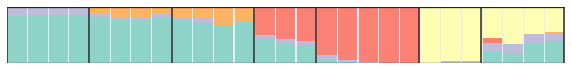In :
structplot("analysis_structure/RADr6.outfile", 6,
colorder=[0,4,2,5,3,1], barorder = [0,1,2,3,4,5])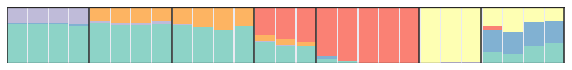In :
structplot("analysis_structure/RADr7.outfile", 7,
colorder=[0,5,6,3,2,4,1], barorder = [0,1,2,3,6,5,4])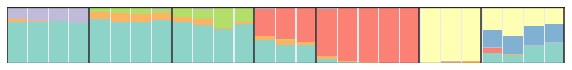In :
structplot("analysis_structure/RADr8.outfile", 8,
colorder=[0,6,3,5,2,7,4,1], barorder = [7,6,4,5,2,0,1,3])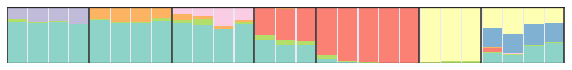### Calculating optimal K¶

The "_f" output files were zipped into the directory as analysis_structure/struct.zip which is in the git repository. This file was uploaded to the online resource Structue Harvester which created the output files that are saved in the git repository in analysis_structure/Harvester.tar.gz. This analysis found K=3 as the optimal K value (highest Delta K) using the Evanno's K method, including the output table below.

In [ ]:
## K	Reps	Mean LnP(K)	Stdev LnP(K)	Ln'(K)	|Ln''(K)|	Delta K
## 2	10	-150180.520000	758.978748	—	—	—
## 3	10	-132621.090000	35.788218	17559.430000	26700.270000	746.063129
## 4	10	-141761.930000	904.565336	-9140.840000	298432.520000	329.918148
## 5	10	-449335.290000	240461.093921	-307573.360000	10843.300000	0.045094
## 6	10	-767751.950000	1281470.488619	-318416.660000	2029640.650000	1.583837
## 7	10	-3115809.260000	2635226.639916	-2348057.310000	344387.090000	0.130686
## 8	10	-5808253.660000	3569473.653017	-2692444.400000	—	—